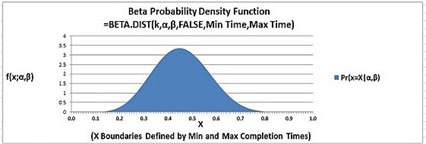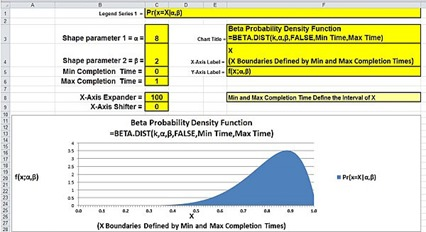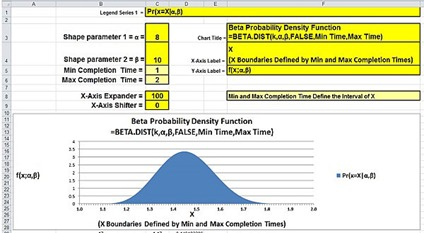## Wednesday, May 28, 2014

### Interactive Graph of the Beta Distribution in Excel 2010 and Excel 2013(Click On Image To See a Larger Version)

This is one of the following eleven articles on creating user-interactive graphs of statistical distributions in Excel

Interactive Statistical Distribution Graph in Excel 2010 and Excel 2013

Interactive Graph of the Normal Distribution in Excel 2010 and Excel 2013

Interactive Graph of the Chi-Square Distribution in Excel 2010 and Excel 2013

Interactive Graph of the t-Distribution in Excel 2010 and Excel 2013

Interactive Graph of the t-Distribution’s PDF in Excel 2010 and Excel 2013

Interactive Graph of the t-Distribution’s CDF in Excel 2010 and Excel 2013

Interactive Graph of the Binomial Distribution in Excel 2010 and Excel 2013

Interactive Graph of the Exponential Distribution in Excel 2010 and Excel 2013

Interactive Graph of the Beta Distribution in Excel 2010 and Excel 2013

Interactive Graph of the Gamma Distribution in Excel 2010 and Excel 2013

Interactive Graph of the Poisson Distribution in Excel 2010 and Excel 2013

# Overview of the Beta Distribution

The beta distribution is a family of continuous probability distributions. This is evidenced by the smooth shape of the above graph of a beta distribution’s PDF (Probability Density Function) curve. The beta distribution is defined on the interval [0,1] and is defined by two positive shape parameters α and β. The Excel formulas that calculate the PDF and CDF of the beta distribution have optional inputs that enable the [0, 1] interval to be expanded to an arbitrary width.

The beta distribution is most often used to model events which are constrained to take place between a minimum and maximum time limit. In this case the beta distribution is used to calculate the probability that a project will be completed within a given period of time. For this reason, the beta distribution is often used for modeling project planning and control systems such as PERT (Project Evaluation and Review Technique) and CPM (Critical Path Method).

The beta distribution is used in general to model the variation of a variable across samples, such as the percentage of the day people spend watching television. The beta distribution can be applied to a wide variety of disciplines to model the behavior of a random variable limited to intervals of finite length. Examples include the modeling of the variability of soil properties and the proportions of the minerals in rocks in stratigraphy.

## Graphing the Distribution’s PDF – Probability Density Function

### Step 1 – Create a Count

The Count becomes the basis for the X and Y values of each data point on the graph. This count will contain 100 points that count from 0 to 100 in increments 1. There are many ways to create a count. This count uses the method ROW() – ROW(\$B) to increment each cell value in the column by 1.(Click On Image To See a Larger Version)

### Step 2 – Create the X Values

One X value is created for each increment of the Count. The Min and Max Completion Times determine how wide the graph will be and therefore are part of the X value calculation.(Click On Image To See a Larger Version)

### Step 3 – Create the Y Values

One Y value is created for each increment of the Count. The Y value of each data point is its PDF value. The beta distribution’s PDF value requires the X value and its four parameters (α, β, and the Min and Max Completion Times) as follows:(Click On Image To See a Larger Version)

The following Excel-generated graph shows the beta distribution’s PDF (Probability Density Function) for as the X value (the completion time) goes from 0 to 1 with α = 8 and β = 10.

The PDF value of a statistical distribution (the Y value) at a specific X value equals the probability that the value of a random sample will be equal to that X value if the population of data values from which the sample was taken is distributed according the stated distribution. The CDF value of a statistical distribution (the Y value) at a specific X value equals the probability that the value of a random sample will be up to that X value.(Click On Image To See a Larger Version)

The process of creating an Excel area chart and connecting the user inputs to the chart is shown in detail in the section of this manual that provides instructions on how to create an interactive normal distribution PDF curve with outer tails.

### The Effect of Changing Shape Parameter α

The following Excel-generated graph shows the Beta distribution’s PDF (Probability Density Function) for as the X value (the completion time) goes from 0 to 1 with α = 4 and β = 10.(Click On Image To See a Larger Version)

### The Effect of Changing Shape Parameter β

The following Excel-generated graph shows the Beta distribution’s PDF (Probability Density Function) for as the X value (the completion time) goes from 0 to 1 with α = 8 and β = 2.(Click On Image To See a Larger Version)

### The Effect of Changing Min and Max Completion Times

The following Excel-generated graph shows the Beta distribution’s PDF (Probability Density Function) as the X value (the completion time) goes from 1 to 2 with α = 8 and β = 10. Note the revised values of the X-axis units.(Click On Image To See a Larger Version)

### The Effect of Shifting and Expanding the X-Axis

The following Excel-generated graph shows effect of shifting and expanding the X-axis as the X value (the completion time) goes from 2 to 5 with α = 8 and β = 2. The X-axis was shifted and expanded in order to optimally present the entire PDF curve in a single graph.(Click On Image To See a Larger Version)

## Graphing the Beta Distribution’s CDF – Cumulative Distribution Function

The following Excel-generated graph shows the Beta distribution’s CDF (Cumulative Distribution Function) for 10 degrees of freedom as the X value (the completion time) goes from a completion time of 0 to 1.

The CDF value of a statistical distribution (the Y value) at a specific X value equals the probability that the value of a random sample will be up to that X value if the population of data values from which the sample was taken is distributed according the stated distribution. The PDF value of a statistical distribution (the Y value) at a specific X value equals the probability that the value of a random sample will be equal to that X value.(Click On Image To See a Larger Version)

Excel Master Series Blog Directory

Statistical Topics and Articles In Each Topic

• Histograms in Excel
• Bar Chart in Excel
• Combinations & Permutations in Excel
• Normal Distribution in Excel
• t-Distribution in Excel
• Binomial Distribution in Excel
• z-Tests in Excel
• t-Tests in Excel
• Hypothesis Tests of Proportion in Excel
• Chi-Square Independence Tests in Excel
• Chi-Square Goodness-Of-Fit Tests in Excel
• F Tests in Excel
• Correlation in Excel
• Pearson Correlation in Excel
• Spearman Correlation in Excel
• Confidence Intervals in Excel
• Simple Linear Regression in Excel
• Multiple Linear Regression in Excel
• Logistic Regression in Excel
• Single-Factor ANOVA in Excel
• Two-Factor ANOVA With Replication in Excel
• Two-Factor ANOVA Without Replication in Excel
• Randomized Block Design ANOVA in Excel
• Repeated-Measures ANOVA in Excel
• ANCOVA in Excel
• Normality Testing in Excel
• Nonparametric Testing in Excel
• Post Hoc Testing in Excel
• Creating Interactive Graphs of Statistical Distributions in Excel
• Solving Problems With Other Distributions in Excel
• Optimization With Excel Solver
• Chi-Square Population Variance Test in Excel
• Analyzing Data With Pivot Tables
• SEO Functions in Excel
• Time Series Analysis in Excel
• VLOOKUP

1.2.